• 霍夫曼树及霍夫曼编码的C语言实现

万次阅读 多人点赞 2016-05-08 21:15:32
现在对学习霍夫曼树的过程加以记录首先介绍霍夫曼树霍夫曼树（Huffman Tree），又称最优二叉树，是一类带权路径长度最短的。假设有n个权值{w1,w2,…,wn}，如果构造一棵有n个叶子节点的二叉树，而这n个叶子节点的...

从周五开始学习霍夫曼树，一直到今天终于完成，期间遇到了各种各样的棘手的问题，通过一遍遍在纸上分析每一步的具体状态得以解决。现在对学习霍夫曼树的过程加以记录

首先介绍霍夫曼树

霍夫曼树（Huffman Tree），又称最优二叉树，是一类带权路径长度最短的树。假设有n个权值{w1,w2,…,wn}，如果构造一棵有n个叶子节点的二叉树，而这n个叶子节点的权值是{w1,w2,…,wn}，则所构造出的带权路径长度最小的二叉树就被称为赫夫曼树。

这里补充下树的带权路径长度的概念。树的带权路径长度指树中所有叶子节点到根节点的路径长度与该叶子节点权值的乘积之和，如果在一棵二叉树中共有n个叶子节点，用Wi表示第i个叶子节点的权值，Li表示第i个也叶子节点到根节点的路径长度，则该二叉树的带权路径长度 WPL=W1*L1 + W2*L2 + … Wn*Ln。

根据节点的个数以及权值的不同，赫夫曼树的形状也各不相同，赫夫曼树具有如下特性：

对于同一组权值，所能得到的霍夫曼树不一定是唯一的。
赫夫曼树的左右子树可以互换，因为这并不影响树的带权路径长度。
带权值的节点都是叶子节点，不带权值的节点都是某棵子二叉树的根节点。
权值越大的节点越靠近赫夫曼树的根节点，权值越小的节点越远离赫夫曼树的根节点。
赫夫曼树中只有叶子节点和度为2的节点，没有度为1的节点。
一棵有n个叶子节点的赫夫曼树共有2n-1个节点。

赫夫曼树的构建步骤如下：
1、将给定的n个权值看做n棵只有根节点（无左右孩子）的二叉树，组成一个集合HT，每棵树的权值为该节点的权值。
2、从集合HT中选出2棵权值最小的二叉树，组成一棵新的二叉树，其权值为这2棵二叉树的权值之和。
3、将步骤2中选出的2棵二叉树从集合HT中删去，同时将步骤2中新得到的二叉树加入到集合HT中。
4、重复步骤2和步骤3，直到集合HT中只含一棵树，这棵树便是赫夫曼树。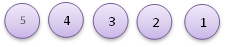假如给定如下5个权值：

则按照以上步骤，可以构造出如下面左图所示的赫夫曼树，当然也可能构造出如下面右图所示的赫夫曼树，这并不是唯一的。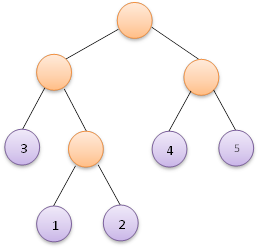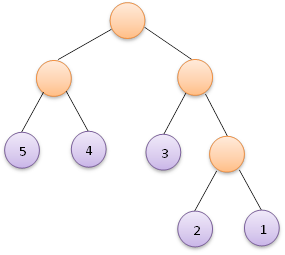Huffman编码
赫夫曼树的应用十分广泛，比如众所周知的在通信电文中的应用。在等传送电文时，我们希望电文的总长尽可能短，因此可以对每个字符设计长度不等的编码，让电文中出现较多的字符采用尽可能短的编码。为了保证在译码时不出现歧义，我们可以采取如下图所示的编码方式：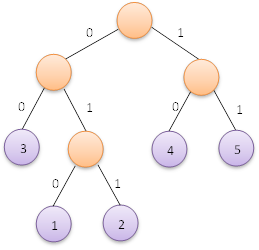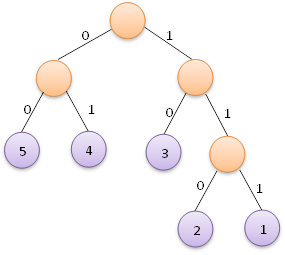即左分支编码为字符0，右分支编码为字符1，将从根节点到叶子节点的路径上分支字符组成的字符串作为叶子节点字符的编码，这便是赫夫曼编码。我们根据上面左图可以得到各叶子节点的赫夫曼编码如下：
权值为5的也自己节点的赫夫曼编码为：11
权值为4的也自己节点的赫夫曼编码为：10
权值为3的也自己节点的赫夫曼编码为：00
权值为2的也自己节点的赫夫曼编码为：011
权值为1的也自己节点的赫夫曼编码为：010

而对于上面右图，则可以得到各叶子节点的赫夫曼编码如下：
权值为5的也自己节点的赫夫曼编码为：00
权值为4的也自己节点的赫夫曼编码为：01
权值为3的也自己节点的赫夫曼编码为：10
权值为2的也自己节点的赫夫曼编码为：110
权值为1的也自己节点的赫夫曼编码为：111

下面给出C语言实现

#include<stdio.h>
#include<stdlib.h>
#include<string.h>
#define OK 1
#define ERROR 0
#define TRUE 1
#define FALSE 0
typedef int Status;
/*定义霍夫曼树节点*/
typedef struct HTNode{
int parent;/*记录双亲*/
int Lchild;/*左右子树*/
int Rchild;
int Weight;/*记录权重*/
}HTNode;
typedef struct HTNode * HuffmanTree;
typedef char ** HuffmanCode;

/*在前k棵树种找到权重最小的树*/
int Min(HuffmanTree HT,int k){
int i=0,min_weight=0,min=0;
/*找出第一个双亲存在的节点，将其权值赋值给min_weight*/
/*注意此处不能直接将HT.weight赋给min_weight，原因是如果HT.weight最小，那么在第一次构造二叉树时就会被选走，而后续的每一轮选择最小权值构造二叉树的比较
还是先用HT.weight的值来进行判断，这样又会再次将其选走，从而产生逻辑上的错误。*/
while(HT[i].parent!=-1)
i++;
min_weight=HT[i].Weight;
min=i;
for(i;i<k;i++){
if(HT[i].Weight<min_weight&&HT[i].parent==-1){
min_weight=HT[i].Weight;
min=i;
}
}
/*找到最小权重的树，将其双亲置为1*/
/*！！！！！注意这的HT的下标！！！！！一晚上才找出这个小问题，别写成HT[i]!!!!!!*/
HT[min].parent=1;
return min;
}

/*从前k棵树中选出权重最小的两棵树,将其序号赋给min1和min2*/
Status SelectMin(HuffmanTree HT,int k,int &min1,int &min2){
min1=Min(HT,k);
min2=Min(HT,k);
return OK;
}

/*创建一课霍夫曼树，-1表示不存在*/
/*wet为一个记录权重的数组，类型为int*/
HuffmanTree CreateHuffmanTree(HuffmanTree HT,int *wet,int n){
int i=0;
int total=2*n-1;/*有n个数据需要编码，即有n个叶子节点，也就有n-1个度为2的节点，总节点数为n+n-1=2*n-1*/
/*初始状态下，前n个节点的双亲，左右子树应该均为-1，权重为对应的权重*/
/*用HT的前n个分量存储n棵树(由n个待编码的数据组成)的森林*/
/*申请total个int组成的动态数组*/
HT=(HuffmanTree)malloc(total*sizeof(HTNode));
if(!HT)
return ERROR;
for(i=0;i<n;i++){
HT[i].Lchild=-1;
HT[i].parent=-1;
HT[i].Rchild=-1;
HT[i].Weight=*wet;
wet++;
}

/*对n到total的分量进行初始化*/
for(i;i<total;i++){
HT[i].Lchild=-1;
HT[i].Rchild=-1;
HT[i].parent=-1;
HT[i].Weight=0;
}
/*用HT的后n-1个分量存储霍夫曼树*/
/*调用函数SelectMin找出森林中两棵权重最小的树*/
int min1=0,min2=0;
for(i=n;i<total;i++){
SelectMin(HT,i,min1,min2);
HT[min1].parent=i;
HT[min2].parent=i;
HT[i].Lchild=min1;
HT[i].Rchild=min2;
HT[i].Weight=HT[min1].Weight+HT[min2].Weight;
}
return HT;
}

/*从叶子节点开始逆向进行霍夫曼编码*/
/*HC用来储存霍夫曼编码值*/
Status HuffmanCoding(HuffmanTree HT,HuffmanCode &HC,int n){
/*HC本身是一个char类型数组的指针,其指向n个char类型的地址，所以给HC分配内存应该写成下面那样*/
HC=(HuffmanCode)malloc(n*sizeof(char *));
if(!HC)
return ERROR;
/*声明一个动态数组code，用来临时存储霍夫曼编码,数组含有n-1个霍夫曼码，加上一个'\0'终止符正好是n个元素，所以分配内存时*/
char *code;
code=(char *)malloc(n*sizeof(char));
if(!code)
return ERROR;
code[n-1]='\0';/*让最后一个元素为终止符*/

int i=0;
for(i=0;i<n;i++){
int current=i;
int father=HT[i].parent;
int start=n-1;
while(father!=-1){
if(current==HT[father].Lchild)
code[--start]='0';
else
code[--start]='1';
current=father;
father=HT[father].parent;
}
/*HC[i]用于最终存储霍夫曼码，是char类型的数组，有n个char类型的数据*/
HC[i]=(char *)malloc((n-start)*sizeof(char));
if(!HC[i])
return ERROR;
/*从临时空间中复制到HC[i]中*/
strcpy(HC[i],code+start);
}
/*释放临时存储空间*/
free(code);
code=NULL;
return OK;
}

int main(void){
int amount=0,i=0;
int *wet=(int *)malloc(amount*sizeof(int));
printf("请输入要编码的字符个数(个数为整型且>1)");
scanf("%d",&amount);
while(amount<=1){
printf("字符个数必须大于1\n");
scanf("%d",&amount);
}
printf("请输入要编码的字符的权值");
for(i=0;i<amount;i++){
scanf("%d",wet+i);
}
HuffmanTree HT;
HT=CreateHuffmanTree(HT,wet,amount);

HuffmanCode HC;
HuffmanCoding(HT,HC,amount);
for(i=0;i<amount;i++){
puts(HC[i]);
}
free(wet);
return OK;
}

2016-05-09更新:
今日在复习霍夫曼树的过程中，发现自己忽视了一些细节问题，造成了一些错误，下面加以记录并反思

问题一:出现在函数Min中

int Min(HuffmanTree HT,int k){
int min=0,min_weight=0,i=0;
/*注意此处是先寻找双亲不存在的节点再赋值，给min_weight赋值应该在循环以外*/
while(HT[i].parent!=-1)
i++;
min_weight=HT[i].weight;
min=i;
for(i;i<k;i++){
if(HT[i].weight<min_weight&&HT[i].parent==-1){
min_weight=HT[i].weight;
min=i;
}
}
/*赋值之后切记要将其双亲赋值为1，否则会出现错误*/
HT[min].parent=1;
return min;
}

问题二:出现在CreateHuffmanTree函数中

HuffmanTree CreateHuffmanTree(HuffmanTree HT,int * wet,int amount){
int i=0,min_weight=0,min=0;
int total=2*amount-1;
/*注意此处分配内存时，sizeof()中应该是HTNode！！要对HT的本质进行理解，HT是一棵树！*/
HT=(HuffmanTree)malloc(total*sizeof(HTNode));
if(!HT)
return ERROR;
for(i=0;i<amount;i++){
HT[i].Lchild=-1;
HT[i].parent=-1;
HT[i].Rchild=-1;
HT[i].weight=*wet;
wet++;
}
for(i;i<total;i++){
HT[i].Lchild=-1;
HT[i].Rchild=-1;
HT[i].parent=-1;
HT[i].weight=0;
}

int min1=0,min2=0;
/*注意此处i的初始值，要对这个过程加以理解，霍夫曼树是用amount之后的分量来存储的，故不能写i=0*/
for(i=amount;i<total;i++){
SelectMin(HT,min1,min2,i);
HT[min1].parent=i;
HT[min2].parent=i;
HT[i].Lchild=min1;
HT[i].Rchild=min2;
HT[i].weight=HT[min1].weight+HT[min2].weight;/*注意新生成的节点的权值为选出的两个最小的节点的权值之和*/
}
return HT;
}

这个问题主要是对建立霍夫曼树的过程理解不够透彻，导致在为HT分配内存时写错大小，和循环过程中将i的初始值误写为0，今后应当注意。

问题三:出现在HuffmanCoding函数中

Status HuffmanCoding(HuffmanTree HT,HuffmanCode &HC,int amount){
int i=0;
/*要先给HC分配内存。HC存储了amount个指向霍夫曼码的指针*/
HC=(HuffmanCode)malloc(amount*sizeof(char *));
if(!HC)
return ERROR;
char * code;
code=(char *)malloc(amount*sizeof(char));
if(!code)
return ERROR;
code[amount-1]='\0';
for(i=0;i<amount;i++){
int current=i;
int start=amount-1;
int father=HT[i].parent;
while(father!=-1){
if(current==HT[father].Lchild)
code[--start]='0';
else
code[--start]='1';
current=father;
father=HT[father].parent;
}
/*HC[i]是HC中若干个存储单元之一，存储着一个具体字符的霍夫曼码，所以分配内存空间时sizeof()中为char*/
HC[i]=(char *)malloc((amount-start)*sizeof(char));
if(!HC[i])
return ERROR;
strcpy(HC[i],code+start);
}
free(code);
code=NULL;
}

对HC的用途理解不够到位，再次强调，HC是一个存储了若干个指向霍夫曼码的指针的数组，HC[i]则用于存储具体字符的霍夫曼码

问题四:反复出现在主函数之中，必须加以记录，认真反省

int main(void){
HuffmanTree HT;
int amount=0;
printf("请输入需要编码的字符个数");
scanf("%d",&amount);
int i=0;
int * wet=(int *)malloc(amount*sizeof(int));
printf("请输入每个字符的权重");
for(i=0;i<amount;i++){
scanf("%d",wet+i);
}
/*此处忘记写HT=，导致未能成功建立霍夫曼树，由于这里要改变函数形参的值，一般情况考虑传入指针变量，但这个函数如果写成指针又太复杂，
很容易出错，故这里使用return把建立好的霍夫曼树直接返回，会方便许多，但是切记要把返回值赋给HT！！！*/
HT=CreateHuffmanTree(HT,wet,amount);
HuffmanCode HC;
HuffmanCoding(HT,HC,amount);
for(i=0;i<amount;i++){
puts(HC[i]);
}
free(wet);
}
展开全文•哈夫曼树
• a，路径和路径长度如果在一棵中有一个节点序列k1，k2，...，kj，因此ki是ki + 1的父代(1 <= i 从k1到kj的分支数称为这两点之间的路径长度，它等于路径上的节点数减去1.b. 节点权重和加权路径长度在许多应用程序...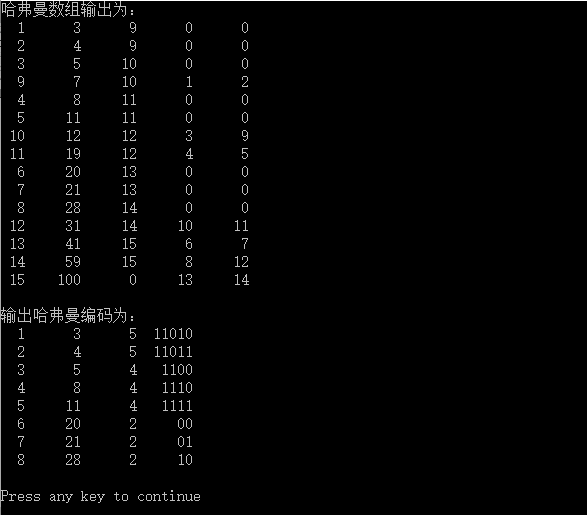a，路径和路径长度

如果在一棵树中有一个节点序列k1，k2，...，kj，因此ki是ki + 1的父代(1 <= i 从k1到kj的分支数称为这两点之间的路径长度，它等于路径上的节点数减去1.

b. 节点权重和加权路径长度

在许多应用程序中，树中的节点通常被赋予具有一定含义的实数. 我们称这个实数为节点的权重(例如下面树中的蓝色数字表示该节点的权重)

节点的加权路径长度定义为从根节点到该节点的路径长度与该节点上的权重的乘积.

c. 树的加权路径长度

树的加权路径长度定义为树中所有叶节点的加权路径长度之和，公式为:

其中，n表示叶节点的数量，wi和li分别表示叶节点ki的权重和从根节点到ki的路径长度.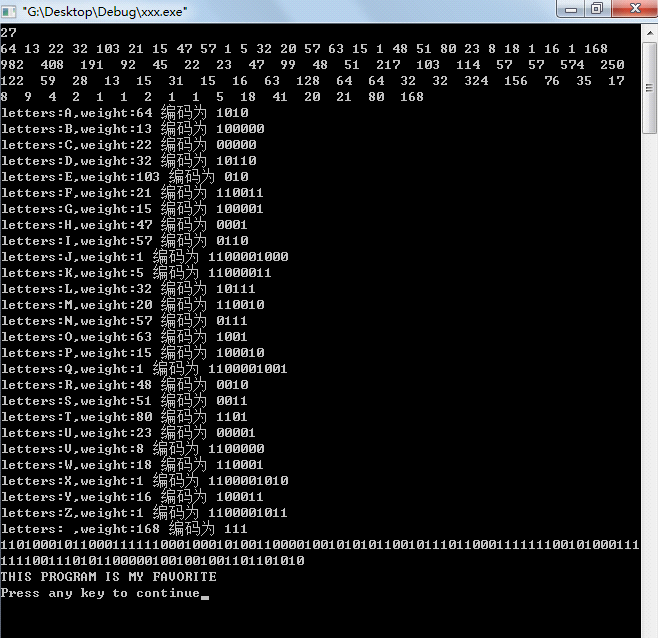下图中的树的加权路径长度WPL = 9 x 2 + 12 x 2 + 15 x 2 + 6 x 3 + 3 x 4 + 5 x 4 = 122

d. 霍夫曼树

霍夫曼树也称为最佳二叉树. 它是由n个加权叶节点组成的所有二叉树中加权路径长度WPL最小的二叉树.

下图是霍夫曼树的.

假设权重为n，则构造的霍夫曼树具有n个叶节点. 将n个权重设置为w1，w2，...，wn，则霍夫曼树的构造规则为:

(1)将w1，w2，...，wn视为n棵树的森林(每棵树只有一个节点)；

(2)在林中，选择要合并的根节点权重最小的两棵树

(3)从森林中删除两棵选定的树，然后将新树添加到森林中；

(4)重复步骤(2)和(3)，直到森林中只剩下一棵树. 这棵树是获得的霍夫曼树.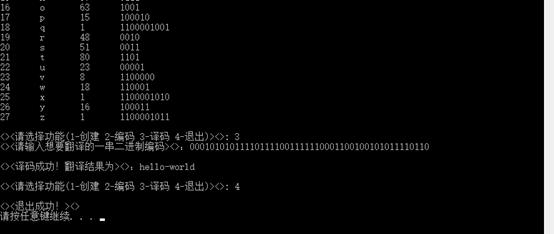例如: 要从下图中的六个加权叶子节点构建霍夫曼树，步骤如下:

注意: 为了使生成的霍夫曼树的结构尽可能唯一，通常指定生成的霍夫曼树中每个节点的左子树根节点的权重小于或等于权重右子树的根节点.

具体算法如下:

/**

* 创建哈夫曼树

*/

PtrHuffman createHuffmanTree(ElemType arr[]){

PtrHuffman ptrArr[LENGTH];

PtrHuffman ptr,pRoot=NULL;

for (int i = ; i < LENGTH; i++){ //初始化结构体指针数组,数组中每一个元素为一个结构体指针类型

ptr = (PtrHuffman)malloc(sizeof(HuffmanTreeNode));

ptr->data = arr[i];

ptr->left = ptr->right = NULL;

ptrArr[i] = ptr;

}

for(i = ; i < LENGTH; i++){ //进行 n-1 次循环建立哈夫曼树

//k1表示森林中具有最小权值的树根结点的下标,k2为次最小的下标

int k1 = -, k2;

for(int j = ; j < LENGTH; j++){

if (ptrArr[j] != NULL && k1 == -){

k1 = j;

continue;

}

if (ptrArr[j] != NULL){

k2 = j;

break;

}

}

//将指针数组中的指针指向的最小值赋值给索引号为k1的,次小值赋值给索引号为k2的

for (j = k2; j < LENGTH; j++){

if(ptrArr[j] != NULL){

if(ptrArr[j]->data < ptrArr[k1]->data){

k2 = k1;

k1 = j;

}else if(ptrArr[j]->data < ptrArr[k2]->data){

k2 = j;

}

}

}

//由最小权值树和次最小权值树建立一棵新树,pRoot指向树根结点

pRoot = (PtrHuffman)malloc(sizeof(HuffmanTreeNode));

pRoot->data = ptrArr[k1]->data + ptrArr[k2]->data;

pRoot->left = ptrArr[k1];

pRoot->right = ptrArr[k2];

ptrArr[k1] = pRoot; //将指向新树的指针赋给ptrArr指针数组中k1位置

ptrArr[k2] = NULL; //k2位置为空

}

return pRoot;

}

在电报通信中，消息以二进制0和1序列传输，每个字符对应一个二进制代码. 为了缩短消息的总长度，采用不等长编码方法构造霍夫曼树.

p>

每个字符的频率作为字符节点的权重被赋予叶节点，每个分支节点的左，右分支分别用0和1编码，从树的根节点到每个叶节点的路径上

分支的0和1编码序列等于叶节点的二进制编码. 上面显示的霍夫曼代码如下:

a的编码为: 00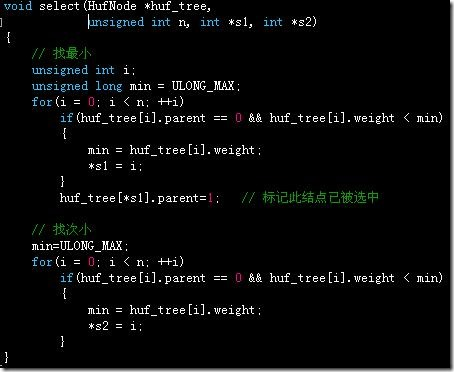b的编码为: 01

c的编码为: 100

d的编码为: 1010

e的编码为: 1011

f的编码为: 11

以上霍夫曼树用作具体示例，以详细的程序演示霍夫曼树的操作:

/** 哈夫曼树编码 **/

#include

#include

#define LENGTH 6

typedef int ElemType;

typedef struct HuffmanTreeNode{

ElemType data; //哈夫曼树中节点的权值

struct HuffmanTreeNode* left;

struct HuffmanTreeNode* right;

}HuffmanTreeNode,*PtrHuffman;

/**

* 创建哈夫曼树

*/

PtrHuffman createHuffmanTree(ElemType arr[]){

PtrHuffman ptrArr[LENGTH];

PtrHuffman ptr,pRoot=NULL;

for (int i = ; i < LENGTH; i++){ //初始化结构体指针数组,数组中每一个元素为一个结构体指针类型

ptr = (PtrHuffman)malloc(sizeof(HuffmanTreeNode));

ptr->data = arr[i];

ptr->left = ptr->right = NULL;

ptrArr[i] = ptr;

}

for(i = ; i < LENGTH; i++){ //进行 n-1 次循环建立哈夫曼树

//k1表示森林中具有最小权值的树根结点的下标,k2为次最小的下标

int k1 = -, k2;

for(int j = ; j < LENGTH; j++){

if (ptrArr[j] != NULL && k1 == -){

k1 = j;

continue;

}

if (ptrArr[j] != NULL){

k2 = j;

break;

}

}

//将指针数组中的指针指向的最小值赋值给索引号为k1的,次小值赋值给索引号为k2的

for (j = k2; j < LENGTH; j++){

if(ptrArr[j] != NULL){

if(ptrArr[j]->data < ptrArr[k1]->data){

k2 = k1;

k1 = j;

}else if(ptrArr[j]->data < ptrArr[k2]->data){

k2 = j;

}

}

}

//由最小权值树和次最小权值树建立一棵新树,pRoot指向树根结点

pRoot = (PtrHuffman)malloc(sizeof(HuffmanTreeNode));

pRoot->data = ptrArr[k1]->data + ptrArr[k2]->data;

pRoot->left = ptrArr[k1];

pRoot->right = ptrArr[k2];

ptrArr[k1] = pRoot; //将指向新树的指针赋给ptrArr指针数组中k1位置

ptrArr[k2] = NULL; //k2位置为空

}

return pRoot;

}

/**

* 计算哈夫曼树带权路径长度WPL

*/

ElemType calculateWeightLength(PtrHuffman &ptrTree,int len){

if(ptrTree==NULL){ //空树返回0

return ;

}else{

if(ptrTree->left==NULL && ptrTree->right==NULL){ //访问到叶子节点

return ptrTree->data * len;

}else{

return calculateWeightLength(ptrTree->left,len+) + calculateWeightLength(ptrTree->right,len+); //向下递归计算

}

}

}

/**

* 哈夫曼树编码(叶子节点按中序方式依次打印其编码)

*/

void HuffmanCoding(PtrHuffman &ptrTree,int len){

//静态局部变量相当于全局变量(只是只有在这个函数中能访问,但是生命周期是和全局变量差不多的)函数退出之后变量还在,而且只在第一次进入的时候做初始化,以后会跳过初始化语句,保留原来的值

static int arr[];

if(ptrTree != NULL){

if(ptrTree->left==NULL && ptrTree->right==NULL){

printf("结点权值为%d的编码: ", ptrTree->data);

for(int i = ; i < len; i++){

printf("%d", arr[i]);

}

printf("\n");

}else{

arr[len] = ;

HuffmanCoding(ptrTree->left,len+);

arr[len] = ;

HuffmanCoding(ptrTree->right,len+);

}

}

}

/**

* 打印哈夫曼树中各个节点的孩子节点

* 若为叶子节点,则只显示提示信息

* @param node 需要显示孩子节点的父节点

*/

void printHuffmanTreeChildNode(PtrHuffman node){

if(node->left == NULL && node->right == NULL){

printf("x=%d是哈夫曼树中的叶子节点",node->data);

printf("\n\n");

return;

}

if(node->left != NULL){

printf("x=%d在哈夫曼树中的左孩子节点是lchild=%d",node->data,node->left->data);

printf("\n");

}

if(node->right != NULL){

printf("x=%d在哈夫曼树中的右孩子节点是rchild=%d",node->data,node->right->data);

printf("\n");

}

printf("\n");

}

/**

* 中序打印哈夫曼树的节点

*/

void midOrderprintHuffmanTreeNode(PtrHuffman &pRoot){

if(pRoot==NULL){

return;

}else{

midOrderprintHuffmanTreeNode(pRoot->left);

printf("%d ",pRoot->data);

midOrderprintHuffmanTreeNode(pRoot->right);

}

}

/**

* 先序打印哈夫曼树的节点

*/

void PreOrderprintHuffmanTreeNode(PtrHuffman &pRoot){

if(pRoot==NULL){

return;

}else{

printHuffmanTreeChildNode(pRoot); //依次打印哈夫曼树中各个节点的孩子节点

PreOrderprintHuffmanTreeNode(pRoot->left);

PreOrderprintHuffmanTreeNode(pRoot->right);

}

}

/**

* 测试程序入口

*/

int main(){

ElemType arr[] = {,,,,,};

PtrHuffman pRoot = createHuffmanTree(arr); //返回指向哈夫曼树根节点的指针

printf("==========中序打印哈夫曼树节点数据==========\n");

midOrderprintHuffmanTreeNode(pRoot);

printf("\n\n");

printf("==========先序打印哈夫曼树节点关系==========\n");

PreOrderprintHuffmanTreeNode(pRoot);

printf("==========计算带权路径长度==========\n");

printf("WeightLength=%d\n",calculateWeightLength(pRoot,));

printf("\n");

printf("==========各节点的哈夫曼树编码==========\n");

HuffmanCoding(pRoot,);

fprintf(stdout,"\n");

return ;

}

运行结果的屏幕截图:

更多有关使用C语言的数据结构实现霍夫曼树的相关文章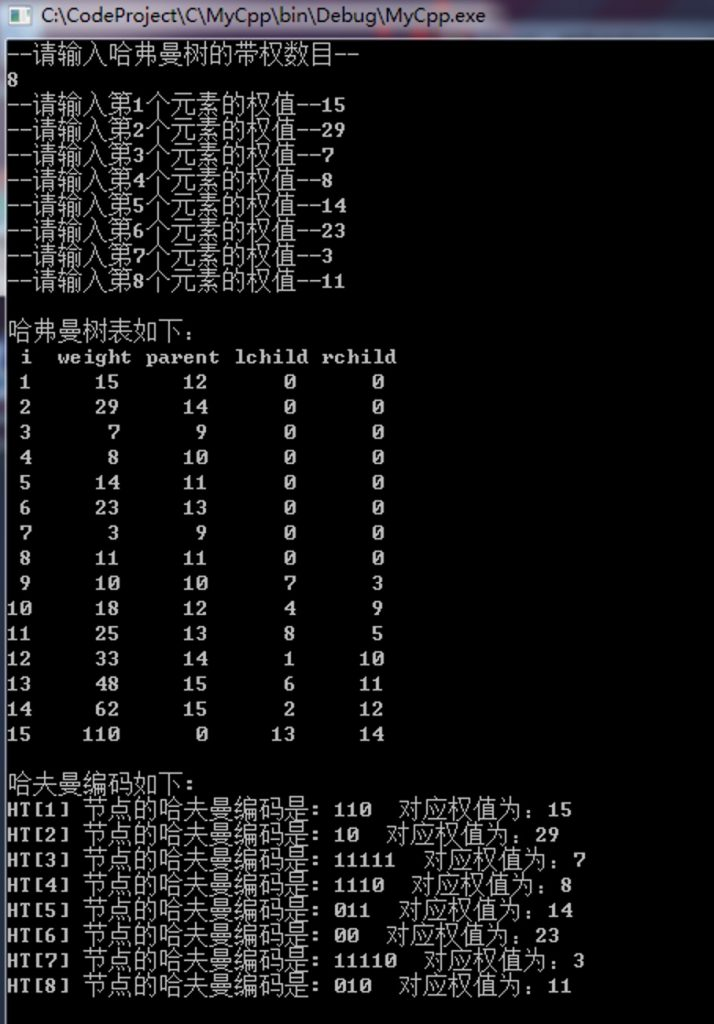0. 数据形分析系列数据结构系列文章数据形分析: 数组. 单链表. 双链表介绍和C ++模板实现数据形分析: 堆栈和C ++模板实现数据结构介绍图形分析: 队列和C ++模板实现的详细说明...

本章介绍霍夫曼树. 与过去一样，本文将首先简要介绍Huffman树的理论知识，然后给出C语言的实现. C ++和Java版本的实现将在后面给出: 实现尽管语言不同，但是原理完全相同，请选择其中一种进行理解. 如果...

上一章介绍了霍夫曼树的基本概念，并在C语言中实现了霍夫曼树. 本章是霍夫曼树的C ++实现. 目录1. Huffman树简介2. Huffman树Manman树的图形分析3. Huffman树的基本操作4.完整的Huffman树源代码已复制...

霍夫曼树分别用C和C ++实现. 本章给出了霍夫曼树的Java版本. 目录1. Huffman树的简介2. Huffman树的图形分析3. Huffman树Man树的基本操作4.再现了Huffman树的完整源代码，请注明出处: htt ..

代码列表如下: #pragma一次#include #include“ stdlib.h” #include ...

版权声明: 本文摘自Wang Lei的博客，未经作者许可，严禁转载. 最近，我在忙于开发新版本. 另外，我正在审查C语言. 这有点慢，但是自从我写了它之后，我一定会尽力组织这部分并分享它...

第6章树和二叉树-教科书的源代码部分中的霍夫曼树(HuffmanTree)--颜为民. 吴维民版源代码使用说明链接

霍夫曼树，由发明人命名，也称为最优树c哈夫曼树编码，是加权路径最短的一种二叉树，主要用于数据压缩传输. 霍夫曼树的构造过程相对比较简单，要了解霍夫曼数，首先必须了解霍夫曼编码. 对于一组不同的频率...

//文件#include #include #include typedef ...

中有QT实现的接口

Java数据结构和算法(4)霍夫曼树的数据结构和算法目录()霍夫曼树也称为最优二叉树，即霍夫曼树...

本文来自电脑杂谈，转载请注明本文网址：

http://www.pc-fly.com/a/jisuanjixue/article-232227-1.html

展开全文c语言霍夫曼函数
• 哈夫曼(霍夫曼树)又称为最优树.1、路径和路径长度在一棵中，从一个结点往下可以达到的孩子或孙子结点之间的通路，称为路径。通路中分支的数目称为路径长度。若规定根结点的层数为1，则从根结点到第L层结点的路径...哈夫曼树(霍夫曼树)又称为最优树.

1、路径和路径长度

在一棵树中，从一个结点往下可以达到的孩子或孙子结点之间的通路，称为路径。通路中分支的数目称为路径长度。若规定根结点的层数为1，则从根结点到第L层结点的路径长度为L-1。

2、结点的权及带权路径长度

若将树中结点赋给一个有着某种含义的数值，则这个数值称为该结点的权。结点的带权路径长度为：从根结点到该结点之间的路径长度与该结点的权的乘积。

3、树的带权路径长度

树的带权路径长度规定为所有叶子结点的带权路径长度之和，记为WPL

#include

#include

#include

/* 哈夫曼树的结构体 */

typedef struct stHuNode

{

int data; //权值

struct stHuNode* lchild, *rchild;

}HUNODE;

/*

* 找出权值数组里面,最小的两个权值下标

* 函数请参：HUNODE *pArray[] 存放节点的指针数组

int n 数组里面的元素个数

int* p1 存放最小权值的下标

int* p2 存放第二小权值的下标

*/

int findSmallData(HUNODE *pArray[] ,int n,int* p1, int* p2)

{

int index = 0;

int fir_small = 0xffff, sec_small = 0xffff;

if(pArray == NULL)

{

return 1;

}

for(index = 0; index < n; index++)

{

/* 当前的下标下面是有节点的*/

if(pArray[index] != NULL)

{

if(pArray[index]->data < fir_small)

{

sec_small = fir_small;

fir_small = pArray[index]->data;

*p2 = *p1;

*p1 = index;

}

else if(pArray[index]->data < sec_small)

{

sec_small = pArray[index]->data;

*p2 = index;

}

}

}

return 0;

}

/*

* 函数功能：构建哈夫曼树

* 函数请参：int* a 权值数组

int n 这个数组里面有多少个数据

*/

HUNODE* createHuTree(int* a, int n)

{

int index = 0;

int fir_small = 0, sec_small = 0;

/* 定义一个指针数组,最大是100 */

HUNODE *pArray;

HUNODE *pNewNode = NULL;

/* 先创建n个root节点*/

memset(pArray,0,sizeof(HUNODE)*n);

for(index = 0; index < n; index++)

{

pNewNode = (HUNODE*)malloc(sizeof(HUNODE));

memset(pNewNode,0,sizeof(HUNODE));

pNewNode->data = a[index];

pNewNode->lchild = NULL;

pNewNode->rchild = NULL;

/* 把这个节点存放在指针数组中去 */

pArray[index] = pNewNode;

}

/* 构建哈夫曼树 */

for(index = 0; index < n-1; index++)

{

/* fir_small 存放最小权值的下标 sec_small存放第二个小的权值下标*/

findSmallData(pArray,n,&fir_small,&sec_small);

/* 分配节点内存 */

pNewNode = (HUNODE*)malloc(sizeof(HUNODE));

memset(pNewNode,0,sizeof(HUNODE));

pNewNode->data = pArray[fir_small]->data + pArray[sec_small]->data;

/* 最小的是左孩子，第二小的是右孩子 */

pNewNode->lchild = pArray[fir_small];

pNewNode->rchild = pArray[sec_small];

/* 把新的节点放入到指针数组里面去 */

pArray[fir_small] = NULL;

pArray[sec_small] = pNewNode;

}

return pNewNode;

}

/* 前序遍历该二叉树 */

void preOrderHuffMan(HUNODE* root)

{

if(root)

{

printf("%d ",root->data);

preOrderHuffMan(root->lchild);

preOrderHuffMan(root->rchild);

}

}

int main()

{

int a = {7,5,2,4};

HUNODE* root = NULL;

/* 构建哈夫曼树 */

root = createHuTree(a,4);

/* 前序遍历 */

preOrderHuffMan(root);

printf("

");

return 0;

}

以上就是本文的全部内容，希望对大家的学习有所帮助，也希望大家多多支持云海天教程。

展开全文• C语言怎么实现哈夫曼构建发布时间：2020-07-29 14:14:08来源：亿速云阅读：98作者：小猪这篇文章主要讲解了C语言怎么实现哈夫曼构建，内容清晰明了，对此有兴趣的小伙伴可以学习一下，相信大家阅读完之后会...

C语言怎么实现哈夫曼树的构建

发布时间：2020-07-29 14:14:08

来源：亿速云

阅读：98

作者：小猪

这篇文章主要讲解了C语言怎么实现哈夫曼树的构建，内容清晰明了，对此有兴趣的小伙伴可以学习一下，相信大家阅读完之后会有帮助。

哈夫曼树(霍夫曼树)又称为最优树.

1、路径和路径长度

在一棵树中，从一个结点往下可以达到的孩子或孙子结点之间的通路，称为路径。通路中分支的数目称为路径长度。若规定根结点的层数为1，则从根结点到第L层结点的路径长度为L-1。

2、结点的权及带权路径长度

若将树中结点赋给一个有着某种含义的数值，则这个数值称为该结点的权。结点的带权路径长度为：从根结点到该结点之间的路径长度与该结点的权的乘积。

3、树的带权路径长度

树的带权路径长度规定为所有叶子结点的带权路径长度之和，记为WPL

#include

#include

#include

/* 哈夫曼树的结构体 */

typedef struct stHuNode

{

int data; //权值

struct stHuNode* lchild, *rchild;

}HUNODE;

/*

* 找出权值数组里面,最小的两个权值下标

* 函数请参：HUNODE *pArray[] 存放节点的指针数组

int n 数组里面的元素个数

int* p1 存放最小权值的下标

int* p2 存放第二小权值的下标

*/

int findSmallData(HUNODE *pArray[] ,int n,int* p1, int* p2)

{

int index = 0;

int fir_small = 0xffff, sec_small = 0xffff;

if(pArray == NULL)

{

return 1;

}

for(index = 0; index < n; index++)

{

/* 当前的下标下面是有节点的*/

if(pArray[index] != NULL)

{

if(pArray[index]->data < fir_small)

{

sec_small = fir_small;

fir_small = pArray[index]->data;

*p2 = *p1;

*p1 = index;

}

else if(pArray[index]->data < sec_small)

{

sec_small = pArray[index]->data;

*p2 = index;

}

}

}

return 0;

}

/*

* 函数功能：构建哈夫曼树

* 函数请参：int* a 权值数组

int n 这个数组里面有多少个数据

*/

HUNODE* createHuTree(int* a, int n)

{

int index = 0;

int fir_small = 0, sec_small = 0;

/* 定义一个指针数组,最大是100 */

HUNODE *pArray;

HUNODE *pNewNode = NULL;

/* 先创建n个root节点*/

memset(pArray,0,sizeof(HUNODE)*n);

for(index = 0; index < n; index++)

{

pNewNode = (HUNODE*)malloc(sizeof(HUNODE));

memset(pNewNode,0,sizeof(HUNODE));

pNewNode->data = a[index];

pNewNode->lchild = NULL;

pNewNode->rchild = NULL;

/* 把这个节点存放在指针数组中去 */

pArray[index] = pNewNode;

}

/* 构建哈夫曼树 */

for(index = 0; index < n-1; index++)

{

/* fir_small 存放最小权值的下标 sec_small存放第二个小的权值下标*/

findSmallData(pArray,n,&fir_small,&sec_small);

/* 分配节点内存 */

pNewNode = (HUNODE*)malloc(sizeof(HUNODE));

memset(pNewNode,0,sizeof(HUNODE));

pNewNode->data = pArray[fir_small]->data + pArray[sec_small]->data;

/* 最小的是左孩子，第二小的是右孩子 */

pNewNode->lchild = pArray[fir_small];

pNewNode->rchild = pArray[sec_small];

/* 把新的节点放入到指针数组里面去 */

pArray[fir_small] = NULL;

pArray[sec_small] = pNewNode;

}

return pNewNode;

}

/* 前序遍历该二叉树 */

void preOrderHuffMan(HUNODE* root)

{

if(root)

{

printf("%d ",root->data);

preOrderHuffMan(root->lchild);

preOrderHuffMan(root->rchild);

}

}

int main()

{

int a = {7,5,2,4};

HUNODE* root = NULL;

/* 构建哈夫曼树 */

root = createHuTree(a,4);

/* 前序遍历 */

preOrderHuffMan(root);

printf("\n");

return 0;

}

看完上述内容，是不是对C语言怎么实现哈夫曼树的构建有进一步的了解，如果还想学习更多内容，欢迎关注亿速云行业资讯频道。

展开全文c语言怎么构建字符树
• 数据结构：C语言实现构建哈夫曼树

万次阅读 多人点赞 2015-08-28 15:08:12
哈夫曼霍夫曼树）又称为最优树.1、路径和路径长度 在一棵中，从一个结点往下可以达到的孩子或孙子结点之间的通路，称为路径。通路中分支的数目称为路径长度。若规定根结点的层数为1，则从根结点到第L层结点的...哈夫曼树 C语言 数据结构
• 1、基本概念a、路径和路径长度若在一棵中存在着一个结点序列 k1，k2，……，kj， 使得 ki是ki+1 的双亲(1<=i从 k1 到 kj 所经过的分支数称为这两点之间的路径长度，它等于路径上的结点数减1.b、结点的权和带权...c语言霍夫曼函数
• 赫夫曼哈夫曼相关的几个名词路径：在一棵中，一个结点到另一个结点之间的通路，称为路径。路径长度：在一条路径中，每经过一个结点，路径长度都要加 1 。例如在一棵中，规定根结点所在层数为1层，那么从根结点...字符串哈夫曼树C语言
• 西安交通大学实验报告课程 数据结构 实验名称 的遍历及霍夫曼树 第 1 页 共 页系别__ 自动化 实 验 日 期 / 2014 年 11 月 22 日专业班级 自动化43班 实 验 报 告 日 期 2014 年 12月 7 日姓名 李欣阳 学号 ...
• 霍夫曼树也称为称最优二叉树，是一种带权路径长度最短的二叉树。所谓的带权路径长度，就是中所有的叶结点的权值乘上其到根结点的路径长度 霍夫曼编码，又译为哈夫曼编码、赫夫曼编码，。是一种用于无损数据压缩...数据结构 哈夫曼树 赫夫曼树 霍夫曼编码 最优二叉树
• *创建哈弗曼 *创建 *每次遍历最小的两个节点 *译码 *遍历（解码的过程） */ #include<stdio.h> #include<stdlib.h> #include<string.h> #define n 4//的叶子节点数 #define m (2*n-1)//...C语言数据结构
• 1、基本概念：霍夫曼(Huffman)又称最优二叉树或最优搜索，是一种带权路径长度最短的二叉树。在许多应用中，常常赋给中结点一个有某种意义的实数，称此实数为该结点的权。从树根结点到该结点之间的路径长度与该...
• 哈夫曼树编码-C语言

千次阅读 多人点赞 2019-11-10 17:02:45
哈夫曼编码 1.实验目的 了解二叉树的定义，理解二叉树的基本性质和存储结构，掌握哈夫曼的构造，实现哈夫曼编码与译码算法。 2.实验内容 从键盘输入一串电文字符与权值，输出对应的哈夫曼编码；从键盘输入一...数据结构 哈夫曼树编码 C语言 算法
• 1、对输入的字符串统计出现频率，进行哈夫曼编码。。...2、生成的哈夫曼编码以及哈夫曼可保存到本地文件。。 3、对接下来输入的01字符串，用先前的哈夫曼编码进行解码。。 4、全过程C语言实现。。
• C语言-哈夫曼树与哈夫曼编码的实现

千次阅读 多人点赞 2020-11-08 00:47:33数据结构 二叉树 算法
• 5.哈夫曼 5.1概念 哈夫曼： 哈夫曼(Huffman )又称最优树，是一类带权路径长度最短的，在实际中有广泛的用途。哈夫曼的定义，涉及路径、路径长度、权等概念，下面先给出这些概念的定义，然后再介绍哈夫曼...数据结构 树结构 霍夫曼树
• //创建哈夫曼 void select(HuffmanTree ht,int n,int *s1,int *s2);//在前n个选项中选权值最小，且双亲为0的两个结点 main() { int n=5; HuffmanTree ht; int w[n]={5,7,3,2,8}; Create_HuffmanTree(ht,w,...
• 哈夫曼的概念以及算法简述 1.相关名次的概念： 路径和路径长度：从中的一个节点到达另外一个节点的之间的分支为路径，其长度为路径长度。的路径长度定义为从根节点开始到达每一个节点的路径长度之和。 权值：...哈夫曼树 数据结构 c语言
• python实现霍夫曼树以及编码

千次阅读 2018-11-29 16:49:08
python实现霍夫曼树以及编码 再看移动通信的时候了解到了霍夫曼（Huffman）编码，花了一些时间进行了霍夫曼编码的python实现。文章内容包括霍夫曼树的生成，以及相应编码的生成，每一部分都会有完整的代码，个人...python 二叉树路径 霍夫曼编码 哈夫曼编码 遍历
• 哈夫曼树和哈夫曼编码详解（C语言实现）

万次阅读 多人点赞 2020-04-15 12:36:48
赫夫曼，别名“哈夫曼”、“最优树”以及“最优二叉树”。学习哈夫曼之前，首先要了解几个名词。 哈夫曼相关的几个名词 路径：在一棵中，一个结点到另一个结点之间的通路，称为路径。图1 中，从根结点到...哈夫曼树 哈夫曼编码 赫夫曼树 赫夫曼编码
• 2. 将字符及对应的权重放入节点（node）中，用链表将各个节点有序的（按权重升序）链接； 3. 实现链表的增、删功能； 4. 遍历链表，将链表的前两个节点中权重相加，生成新节点，然后将新节点插入到有序链表中； 5....哈夫曼树
• #include #include #include #include #define OK 1#define ERROR 0#define OVERFLOW -1#define Status int//哈夫曼节点类型定义typedef struct HTNodew{unsigned int weig...
• 用户键盘输入若干个整数作为待编码字符的权值，程序建立哈夫曼并输出各字符的哈夫曼编码。c语言...

构建霍夫曼树c语言c语言 订阅